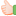1. ##Think and Answer

If X gives Y seven apples, then Y will then have five times as many as X would have, however, if Y give X seven apples, then both X and Y will have the same number of apples. How many apples do X currently have?Reply With Quote

2. ## Re: Think and Answer

x=14
y = 28Reply With Quote

3. ##Re: Think and Answer

Let no of apples with x as n1
Let no of apples with y as n2

Then according to first condition
n2+7 = 5* (n1-7)-----------------------(1)

From the second condition we have
n2-7=n1+7-----------------------------(2)

Thus solving (1) and (2) we have
n1=14 n2=28Reply With Quote

4. ##Re: Think and Answer

Five girls took part in a race. Alison finished before Bunty but behind Clare. Debby finished before Emma but behind Bunty. What was the finishing order?Reply With Quote

5. ##Re: Think and Answer

Finishing Order Is

1.Clare
2.Alison
3.Bunty
4.Debby
5.EmmaReply With Quote

6. ## Re: Think and Answer

Call No. of apple of Y is n1
Call No. of apple of X is n2
We have :

n1 + 5 = 5*(n2 - 5) => n1 = 5*n2 - 30 (1)

on the other hand :

n1 - 7 = n2 + 7 => n1 = n2 + 14 (2)

From (1) and (2), we have

n1 = 25
n2 = 11

I'm sure this is the correct answer.

14 & 28 is not the correct answer.Reply With Quote

####Posting Permissions

• You may not post new threads
• You may not post replies
• You may not post attachments
• You may not edit your posts
•